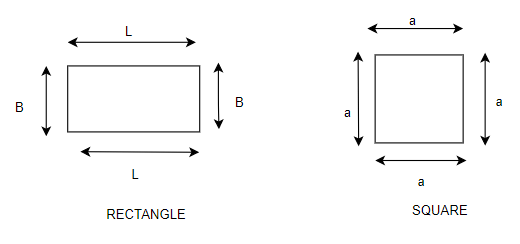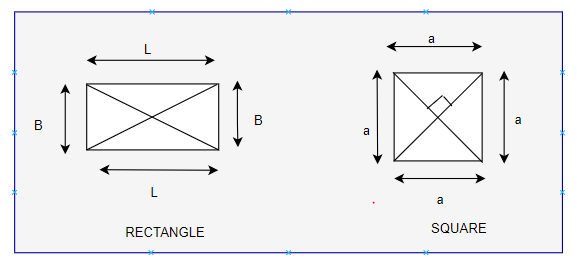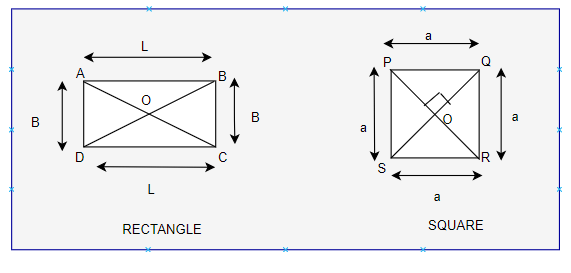Home » Maths » Difference Between Square and Rectangle

# Difference Between Square and Rectangle

The square and rectangle are both members of the Quadrilateral family of four-sided polygons. They have some similarities as well as differences. They both have 90° angles with all angles being equal. In addition, the diagonals are equal.

This article will explain the fundamental difference between squares and rectangles. We’ll also consider the question, “Is a square a rectangle?” Along with learning the differences between square and rectangle, we will also discover the similarities between these two geometrical figures.

## Difference Between Square and Rectangle

1. All sides are of equal length in the Square while Only the opposite sides are equal in the rectangle2. Diagonals of a square are perpendicular bisectors of each other while Diagonals of a rectangle are not perpendicular bisectors of each other3. Diagonals bisect the angles in the square while it does not bisect in a rectangleFor rectangle : $\angle OAB \ne \angle OAD$
For square : $\angle OPQ = \angle OPS$
4. The perimeter of the Square is given by
$P =4a$
The perimeter of the Rectangle is given by
$P =2(L +B)$
5. The area of the Square is given by
$P =a^2$
The area of the Rectangle is given by
$P =2(L +B)$

## Similarities between them

Let us look at the similarities between a square and a rectangle

• All four sides of both square and rectangle are straight.
• Their opposite sides are exactly the same length.
• Their interior angles add up to 360 degrees.
• Their interior angles are all 90 degrees.
• The area of both square and a rectangle is equal to the product of a pair of adjacent sides.

## Is a square a rectangle?

A square is a rectangle, so the answer is yes. A square is a rectangle because it has all of the characteristics of a rectangle.

These characteristics are:

• Each interior angle is 90 degrees.
• Parallel and equal sides on opposite sides
• Two equal diagonals that bisect each other.
Subscribe
Notify of

This site uses Akismet to reduce spam. Learn how your comment data is processed.

1 Comment
Inline Feedbacks